SLOS190H February   1997  – March 2016

PRODUCTION DATA.

1. Features
2. Applications
3. Description
4. Revision History
5. Pin Configuration and Functions
6. Specifications
7. Detailed Description
8. Application and Implementation
1. 8.1 Application Information
2. 8.2 Typical Application
1. 8.2.1 High-Side Current Monitor
9. Power Supply Recommendations
10. 10Layout
11. 11Device and Documentation Support
12. 12Mechanical, Packaging, and Orderable Information

• D|8
• PW|8
• P|8
• D|8

## 6 Specifications

### 6.1 Absolute Maximum Ratings

over operating free-air temperature range (unless otherwise noted)(1)
MIN MAX UNIT
Supply voltage, VDD+(2) 8 V
VDD-(2) –8 V
Differential input voltage, VID(3) ±16 V
Input voltage, VI(any input)(2) VDD− − 0.3 VDD+ V
Input current, II (any input) ±5 mA
Output current, IO ±50 mA
Total current into VDD+ ±50 mA
Total current out of VDD– ±50 mA
Duration of short-circuit current at (or below) 25°C(4) Unlimited
Operating free-air temperature range, TA C level parts 0 70 °C
I, Q level parts –40 125
M level parts –55 125
Lead temperature 1,6 mm (1/16 inch) from case for 10 seconds D, N, P or PW package 260 °C
Lead temperature 1,6 mm (1/16 inch) from case for 60 seconds J or U package 300 °C
Storage temperature, Tstg –65 150 °C
(1) Stresses beyond those listed under Absolute Maximum Ratings may cause permanent damage to the device. These are stress ratings only, which do not imply functional operation of the device at these or any other conditions beyond those indicated under Recommended Operating Conditions. Exposure to absolute-maximum-rated conditions for extended periods may affect device reliability.
(2) All voltage values, except differential voltages, are with respect to the midpoint between VDD+ and VDD.
(3) Differential voltages are at IN+ with respect to IN–. Excessive current will flow if input is brought below VDD– − 0.3 V.
(4) The output may be shorted to either supply. Temperature or supply voltages must be limited to ensure that the maximum dissipation rating is not exceeded.

### 6.2 ESD Ratings

VALUE UNIT
V(ESD) Electrostatic discharge Human-body model (HBM), per AEC Q100-002(1) Q-grade and M-grade devices in D and PW packages ±2000 V
Charged-device model (CDM), per AEC Q100-011 Q-grade and M-grade devices in D and PW packages ±1000
(1) AEC Q100-002 indicates that HBM stressing shall be in accordance with the ANSI/ESDA/JEDEC JS-001 specification.

### 6.3 Recommended Operating Conditions

MIN MAX UNIT
VDD± Supply voltage C LEVEL PARTS ±2.2 ±8 V
I LEVEL PARTS ±2.2 ±8
Q LEVEL PARTS ±2.2 ±8
M LEVEL PARTS ±2.2 ±8
VI Input voltage C LEVEL PARTS VDD− VDD+ −1.5 V
I LEVEL PARTS VDD− VDD+ −1.5
Q LEVEL PARTS VDD− VDD+ −1.5
M LEVEL PARTS VDD− VDD+ −1.5
VIC Common-mode input voltage C LEVEL PARTS VDD− VDD+ −1.5 V
I LEVEL PARTS VDD− VDD+ −1.5
Q LEVEL PARTS VDD− VDD+ −1.5
M LEVEL PARTS VDD− VDD+ −1.5
TA Operating free-air temperature C LEVEL PARTS 0 70 °C
I LEVEL PARTS –40 125
Q LEVEL PARTS –40 125
M LEVEL PARTS –55 125

### 6.4 Thermal Information

THERMAL METRIC(1) TLC2272 TLC2274 UNIT
D
(SOIC)
P
(PDIP)
PW
(TSSOP)
FK
(LCCC)
U
(CFP)
D
(SOIC)
N
(PDIP)
PW
(TSSOP)
FK
(LCCC)
J
(CDIP)
8-PIN 8-PIN 8-PIN 20-PIN 10-PIN 14-PIN 14-PIN 14-PIN 20-PIN 14-PIN
RθJA Junction-to-ambient thermal resistance (2)(3) 115.6 58.5 175.8 83.8 111.6 °C/W
RθJC(top) Junction-to-case (top) thermal resistance (2)(3) 61.8 48.3 58.8 18 121.3 43.2 34 41.2 16 16.2 °C/W
RθJB Junction-to-board thermal resistance 55.9 35.6 104.3 38.4 54.7 °C/W
ψJT Junction-to-top characterization parameter 14.3 25.9 5.9 9.4 3.9 °C/W
ψJB Junction-to-board characterization parameter 55.4 35.5 102.6 38.1 53.9 °C/W
RθJC(bot) Junction-to-case (bottom) thermal resistance 8.68 °C/W
(2) Maximum power dissipation is a function of TJ(max), θJA, and TA. The maximum allowable power dissipation at any allowable ambient temperature is PD = (TJ(max) − TA) / θJA. Operating at the absolute maximum TJ of 150°C can affect reliability.
(3) The package thermal impedance is calculated in accordance with JESD 51-7 (plastic) or MIL-STD-883 Method 1012 (ceramic).

### 6.5 TLC2272 and TLC2272A Electrical Characteristics VDD = 5 V

at specified free-air temperature, VDD = 5 V; TA = 25°C, unless otherwise noted.
PARAMETER TEST CONDITIONS MIN TYP MAX UNIT
VIO Input offset voltage VIC = 0 V, VDD± = ±2.5 V,
VO = 0 V, RS = 50 Ω
TLC2272 TA = 25°C 300 2500 µV
TLC2272A 300 950
TLC2272 Full Range(1) 3000
TLC2272A 1500
αVIO Temperature coefficient of
input offset voltage
VIC = 0 V, VDD± = ±2.5 V, VO = 0 V, RS = 50 Ω 2 μV/°C
Input offset voltage long-term drift(3) VIC = 0 V, VDD± = ±2.5 V, VO = 0 V, RS = 50 Ω 0.002 μV/mo
IIO Input offset current VIC = 0 V, VDD± = ±2.5 V,
VO = 0 V, RS = 50 Ω
All level parts TA = 25°C 0.5 60 pA
C level part TA = 0°C to 80°C 100
I level part TA = –40°C to 85°C 150
Q level part TA = –40°C to 125°C 800
M level part TA = –55°C to 125°C 800
IIB Input bias current VIC = 0 V, VDD± = ±2.5 V,
VO = 0 V, RS = 50 Ω
All level parts TA = 25°C 1 60 pA
C level part TA = 0°C to 80°C 100
I level part TA = –40°C to 85°C 150
Q level part TA = –40°C to 125°C 800
M level part TA = –55°C to 125°C 800
VICR Common-mode input voltage RS = 50 Ω; |VIO | ≤ 5 mV TA = 25°C –0.3 2.5 4 V
Full Range(1) 0 2.5 3.5
VOH High-level output voltage IOH = −20 μA 4.99 V
IOH = −200 μA TA = 25°C 4.85 4.93
Full Range(1) 4.85
IOH = −1 mA TA = 25°C 4.25 4.65
Full Range(1) 4.25
VOL Low-level output voltage VIC = 2.5 V IOL = 50 μA 0.01 V
IOL = 500 μA TA = 25°C 0.09 0.15
Full Range(1) 0.15
IOL = 5 mA TA = 25°C 0.9 1.5
Full Range(1) 1.5
AVD Large-signal differential
voltage amplification
VIC = 2.5 V,
VO = 1 V to 4 V;
RL = 10 kΩ(2)
C level part TA = 25°C 15 35 V/mV
TA = 0°C to 80°C 15
I level part TA = 25°C 15 35
TA = –40°C to 85°C 15
Q level part TA = 25°C 10 35
TA = –40°C to 125°C 10
M level part TA = 25°C 10 35
TA = –55°C to 125°C 10
VIC = 2.5 V, VO = 1 V to 4 V; RL = 1 MΩ(2) 175
rid Differential input resistance 1012 Ω
ri Common-mode input resistance 1012 Ω
ci Common-mode input capacitance f = 10 kHz, P package 8 pF
zo Closed-loop output impedance f = 1 MHz, AV = 10 140 Ω
CMRR Common-mode rejection ratio VIC = 0 V to 2.7 V,
VO = 2.5 V, RS = 50 Ω
TA = 25°C 70 75 dB
Full Range(1) 70
kSVR Supply-voltage rejection ratio
(ΔVDD / ΔVIO)
VDD = 4.4 V to 16 V,
VIC = VDD / 2, no load
TA = 25°C 80 95 dB
Full Range(1) 80
IDD Supply currrent VO = 2.5 V, no load TA = 25°C 2.2 3 mA
Full Range(1) 3
SR Slew rate at unity gain VO = 0.5 V to 2.5 V,
RL = 10 kΩ(2), CL = 100 pF(2)
TA = 25°C 2.3 3.6 V/µs
Full Range(1) 1.7
Vn Equivalent input noise voltage f = 10 Hz 50 nV/√Hz
f = 1 kHz 9
VNPP Peak-to-peak equivalent
input noise voltage
f = 0.1 Hz to 1 Hz 1 µV
f = 0.1 Hz to 10 Hz 1.4
In Equivalent input noise current 0.6 fA/√Hz
THD+N Total harmonic distortion + noise VO = 0.5 V to 2.5 V,
f = 20 kHz, RL = 10 kΩ(2)
AV = 1 0.0013%
AV = 10 0.004%
AV = 100 0.03%
Gain-bandwidth product f = 10 kHz, RL = 10 kΩ(2), CL = 100 pF(2) 2.18 MHz
BOM Maximum output-swing bandwidth VO(PP) = 2 V, AV = 1, RL = 10 kΩ(2), CL = 100 pF(2) 1 MHz
ts Settling time AV = –1, RL = 10 kΩ(2),
Step = 0.5 V to 2.5 V, CL = 100 pF(2)
To 0.1% 1.5 µs
To 0.01% 2.6
φm Phase margin at unity gain RL = 10 kΩ(2), CL = 100 pF(2) 50°
Gain margin RL = 10 kΩ(2), CL = 100 pF(2) 10 dB
(1) TA = –55°C to 125°C.
(2) Referenced to 0 V.
(3) Typical values are based on the input offset voltage shift observed through 168 hours of operating life test at TA = 150°C extrapolated to TA = 25°C using the Arrhenius equation and assuming an activation energy of 0.96 eV.

### 6.6 TLC2272 and TLC2272A Electrical Characteristics VDD± = ±5 V

at specified free-air temperature, VDD± = ±5 V; TA = 25°C, unless otherwise noted.
PARAMETER TEST CONDITIONS MIN TYP MAX UNIT
VIO Input offset voltage VIC = 0 V, VO = 0 V,
RS = 50 Ω
TLC2272 TA = 25°C 300 2500 µV
TLC2272A 300 950
TLC2272 Full Range(1) 3000
TLC2272A 1500
αVIO Temperature coefficient of
input offset voltage
VIC = 0 V, VO = 0 V, RS = 50 Ω 2 μV/°C
Input offset voltage long-term drift(3) VIC = 0 V, VO = 0 V, RS = 50 Ω 0.002 μV/mo
IIO Input offset current VIC = 0 V, VO = 0 V,
RS = 50 Ω
All level parts TA = 25°C 0.5 60 pA
C level part TA = 0°C to 80°C 100
I level part TA = –40°C to 85°C 150
Q level part TA = –40°C to 125°C 800
M level part TA = –55°C to 125°C 800
IIB Input bias current VIC = 0 V, VO = 0 V,
RS = 50 Ω
All level parts TA = 25°C 1 60 pA
C level part TA = 0°C to 80°C 100
I level part TA = –40°C to 85°C 150
Q level part TA = –40°C to 125°C 800
M level part TA = –55°C to 125°C 800
VICR Common-mode input voltage RS = 50 Ω; |VIO | ≤ 5 mV TA = 25°C –5.3 0 4 V
Full Range(1) –5 0 3.5
VOM+ Maximum positive peak
output voltage
IO = −20 μA 4.99 V
IO = −200 μA TA = 25°C 4.85 4.93
Full Range(1) 4.85
IO = −1 mA TA = 25°C 4.25 4.65
Full Range(1) 4.25
VOM- Maximum negative
peak output voltage
VIC = 0 V, IO = 50 μA –4.99 V
IO = 500 μA TA = 25°C –4.85 –4.91
Full Range(1) –4.85
IO = 5 mA TA = 25°C –3.5 –4.1
Full Range(1) –3.5
AVD Large-signal differential
voltage amplification
VO = ±4 V; RL = 10 kΩ C level part TA = 25°C 25 50 V/mV
TA = 0°C to 80°C 25
I level part TA = 25°C 25 50
TA = –40°C to 85°C 25
Q level part TA = 25°C 20 50
TA = –40°C to 125°C 20
M level part TA = 25°C 20 50
TA = –55°C to 125°C 20
VO = ±4 V; RL = 1 MΩ 300
rid Differential input resistance 1012 Ω
ri Common-mode input resistance 1012 Ω
ci Common-mode input capacitance f = 10 kHz, P package 8 pF
zo Closed-loop output impedance f = 1 MHz, AV = 10 130 Ω
CMRR Common-mode rejection ratio VIC = –5 V to 2.7 V,
VO = 0 V, RS = 50 Ω
TA = 25°C 75 80 dB
Full Range(1) 75
kSVR Supply-voltage rejection ratio
(ΔVDD / ΔVIO)
VDD+ = 2.2 V to ±8 V,
VIC = 0 V, no load
TA = 25°C 80 95 dB
Full Range(1) 80
IDD Supply currrent VO = 0 V, no load TA = 25°C 2.4 3 mA
Full Range(1) 3
SR Slew rate at unity gain VO = ±2.3 V,
RL = 10 kΩ, CL = 100 pF
TA = 25°C 2.3 3.6 V/µs
Full Range(1) 1.7
Vn Equivalent input noise voltage f = 10 Hz 50 nV/√Hz
f = 1 kHz 9
VNPP Peak-to-peak equivalent
input noise voltage
f = 0.1 Hz to 1 Hz 1 µV
f = 0.1 Hz to 10 Hz 1.4
In Equivalent input noise current 0.6 fA/√Hz
THD+N Total harmonic distortion + noise VO = ±2.3,
f = 20 kHz, RL = 10 kΩ
AV = 1 0.0011%
AV = 10 0.004%
AV = 100 0.03%
Gain-bandwidth product f = 10 kHz, RL = 10 kΩ, CL = 100 pF 2.25 MHz
BOM Maximum output-swing bandwidth VO(PP) = 4.6 V, AV = 1, RL = 10 kΩ, CL = 100 pF 0.54 MHz
ts Settling time AV = –1, RL = 10 kΩ,
Step = –2.3 V to 2.3 V, CL = 100 pF
To 0.1% 1.5 µs
To 0.01% 3.2
φm Phase margin at unity gain RL = 10 kΩ, CL = 100 pF 52°
Gain margin RL = 10 kΩ, CL = 100 pF 10 dB
(1) TA = –55°C to 125°C.

### 6.7 TLC2274 and TLC2274A Electrical Characteristics VDD = 5 V

at specified free-air temperature, VDD = 5 V; TA = 25°C, unless otherwise noted.
PARAMETER TEST CONDITIONS MIN TYP MAX UNIT
VIO Input offset voltage VIC = 0 V, VDD± = ±2.5 V,
VO = 0 V, RS = 50 Ω
TLC2274 TA = 25°C 300 2500 µV
TLC2274A 300 950
TLC2274 Full Range(1) 3000
TLC2274A 1500
αVIO Temperature coefficient of
input offset voltage
VIC = 0 V, VDD± = ±2.5 V, VO = 0 V, RS = 50 Ω 2 μV/°C
Input offset voltage long-term drift(3) VIC = 0 V, VDD± = ±2.5 V, VO = 0 V, RS = 50 Ω 0.002 μV/mo
IIO Input offset current VIC = 0 V, VDD± = ±2.5 V,
VO = 0 V, RS = 50 Ω
All level parts TA = 25°C 0.5 60 pA
C level part TA = 0°C to 80°C 100
I level part TA = –40°C to 85°C 150
Q level part TA = –40°C to 125°C 800
M level part TA = –55°C to 125°C 800
IIB Input bias current VIC = 0 V, VDD± = ±2.5 V,
VO = 0 V, RS = 50 Ω
All level parts TA = 25°C 1 60 pA
C level part TA = 0°C to 80°C 100
I level part TA = –40°C to 85°C 150
Q level part TA = –40°C to 125°C 800
M level part TA = –55°C to 125°C 800
VICR Common-mode input voltage RS = 50 Ω; |VIO | ≤ 5 mV TA = 25°C –0.3 2.5 4 V
Full Range(1) 0 2.5 3.5
VOH High-level output voltage IOH = −20 μA 4.99 V
IOH = −200 μA TA = 25°C 4.85 4.93
Full Range(1) 4.85
IOH = −1 mA TA = 25°C 4.25 4.65
Full Range(1) 4.25
VOL Low-level output voltage VIC = 2.5 V IOL = 50 μA 0.01 V
IOL = 500 μA TA = 25°C 0.09 0.15
Full Range(1) 0.15
IOL = 5 mA TA = 25°C 0.9 1.5
Full Range(1) 1.5
AVD Large-signal differential
voltage amplification
VIC = 2.5 V, VO = 1 V to 4 V;
RL = 10 kΩ(2)
C level part TA = 25°C 15 35 V/mV
TA = 0°C to 80°C 15
I level part TA = 25°C 15 35
TA = –40°C to 85°C 15
Q level part TA = 25°C 10 35
TA = –40°C to 125°C 10
M level part TA = 25°C 10 35
TA = –55°C to 125°C 10
VIC = 2.5 V, VO = 1 V to 4 V; RL = 1 MΩ(2) 175
rid Differential input resistance 1012 Ω
ri Common-mode input resistance 1012 Ω
ci Common-mode input capacitance f = 10 kHz, P package 8 pF
zo Closed-loop output impedance f = 1 MHz, AV = 10 140 Ω
CMRR Common-mode rejection ratio VIC = 0 V to 2.7 V,
VO = 2.5 V, RS = 50 Ω
TA = 25°C 70 75 dB
Full Range(1) 70
kSVR Supply-voltage rejection ratio
(ΔVDD / ΔVIO)
VDD = 4.4 V to 16 V,
VIC = VDD / 2, no load
TA = 25°C 80 95 dB
Full Range(1) 80
IDD Supply currrent VO = 2.5 V, no load TA = 25°C 4.4 6 mA
Full Range(1) 6
SR Slew rate at unity gain VO = 0.5 V to 2.5 V,
RL = 10 kΩ(2), CL = 100 pF(2)
TA = 25°C 2.3 3.6 V/µs
Full Range(1) 1.7
Vn Equivalent input noise voltage f = 10 Hz 50 nV/√Hz
f = 1 kHz 9
VNPP Peak-to-peak equivalent
input noise voltage
f = 0.1 Hz to 1 Hz 1 µV
f = 0.1 Hz to 10 Hz 1.4
In Equivalent input noise current 0.6 fA/√Hz
THD+N Total harmonic distortion + noise VO = 0.5 V to 2.5 V,
f = 20 kHz, RL = 10 kΩ(2)
AV = 1 0.0013%
AV = 10 0.004%
AV = 100 0.03%
Gain-bandwidth product f = 10 kHz, RL = 10 kΩ(2), CL = 100 pF(2) 2.18 MHz
BOM Maximum output-swing bandwidth VO(PP) = 2 V, AV = 1, RL = 10 kΩ(2), CL = 100 pF(2) 1 MHz
ts Settling time AV = –1, RL = 10 kΩ(2),
Step = 0.5 V to 2.5 V, CL = 100 pF(2)
To 0.1% 1.5 µs
To 0.01% 2.6
φm Phase margin at unity gain RL = 10 kΩ(2), CL = 100 pF(2) 50°
Gain margin RL = 10 kΩ(2), CL = 100 pF(2) 10 dB
(1) TA = –55°C to 125°C.

### 6.8 TLC2274 and TLC2274A Electrical Characteristics VDD± = ±5 V

at specified free-air temperature, VDD± = ±5 V; TA = 25°C, unless otherwise noted.
PARAMETER TEST CONDITIONS MIN TYP MAX UNIT
VIO Input offset voltage VIC = 0 V, VO = 0 V,
RS = 50 Ω
TLC2274 TA = 25°C 300 2500 µV
TLC2274A 300 950
TLC2274 Full Range(1) 3000
TLC2274A 1500
αVIO Temperature coefficient of
input offset voltage
VIC = 0 V, VO = 0 V, RS = 50 Ω 2 μV/°C
Input offset voltage long-term drift(3) VIC = 0 V, VO = 0 V, RS = 50 Ω 0.002 μV/mo
IIO Input offset current VIC = 0 V, VO = 0 V,
RS = 50 Ω
All level parts TA = 25°C 0.5 60 pA
C level part TA = 0°C to 80°C 100
I level part TA = –40°C to 85°C 150
Q level part TA = –40°C to 125°C 800
M level part TA = –55°C to 125°C 800
IIB Input bias current VIC = 0 V, VO = 0 V,
RS = 50 Ω
All level parts TA = 25°C 1 60 pA
C level part TA = 0°C to 80°C 100
I level part TA = –40°C to 85°C 150
Q level part TA = –40°C to 125°C 800
M level part TA = –55°C to 125°C 800
VICR Common-mode input voltage RS = 50 Ω; |VIO | ≤ 5 mV TA = 25°C –5.3 0 4 V
Full Range(1) –5 0 3.5
VOM+ Maximum positive peak
output voltage
IO = −20 μA 4.99 V
IO = −200 μA TA = 25°C 4.85 4.93
Full Range(1) 4.85
IO = −1 mA TA = 25°C 4.25 4.65
Full Range(1) 4.25
VOM- Maximum negative peak
output voltage
VIC = 0 V IO = 50 μA –4.99 V
IO = 500 μA TA = 25°C –4.85 –4.91
Full Range(1) –4.85
IO = 5 mA TA = 25°C –3.5 –4.1
Full Range(1) –3.5
AVD Large-signal differential
voltage amplification
VO = ±4 V; RL = 10 kΩ C level part TA = 25°C 25 50 V/mV
TA = 0°C to 80°C 25
I level part TA = 25°C 25 50
TA = –40°C to 85°C 25
Q level part TA = 25°C 20 50
TA = –40°C to 125°C 20
M level part TA = 25°C 20 50
TA = –55°C to 125°C 20
VO = ±4 V; RL = 1 MΩ 300
rid Differential input resistance 1012 Ω
ri Common-mode input resistance 1012 Ω
ci Common-mode input capacitance f = 10 kHz, P package 8 pF
zo Closed-loop output impedance f = 1 MHz, AV = 10 130 Ω
CMRR Common-mode rejection ratio VIC = –5 V to 2.7 V,
VO = 0 V, RS = 50 Ω
TA = 25°C 75 80 dB
Full Range(1) 75
kSVR Supply-voltage rejection ratio
(ΔVDD / ΔVIO)
VDD+ = 2.2 V to ±8 V,
VIC = 0 V, no load
TA = 25°C 80 95 dB
Full Range(1) 80
IDD Supply currrent VO = 0 V, no load TA = 25°C 4.8 6 mA
Full Range(1) 6
SR Slew rate at unity gain VO = ±2.3 V,
RL = 10 kΩ, CL = 100 pF
TA = 25°C 2.3 3.6 V/µs
Full Range(1) 1.7
Vn Equivalent input noise voltage f = 10 Hz 50 nV/√Hz
f = 1 kHz 9
VNPP Peak-to-peak equivalent
input noise voltage
f = 0.1 Hz to 1 Hz 1 µV
f = 0.1 Hz to 10 Hz 1.4
In Equivalent input noise current 0.6 fA/√Hz
THD+N Total harmonic distortion + noise VO = ±2.3,
f = 20 kHz, RL = 10 kΩ
AV = 1 0.0011%
AV = 10 0.004%
AV = 100 0.03%
Gain-bandwidth product f = 10 kHz, RL = 10 kΩ, CL = 100 pF 2.25 MHz
BOM Maximum output-swing bandwidth VO(PP) = 4.6 V, AV = 1, RL = 10 kΩ, CL = 100 pF 0.54 MHz
ts Settling time AV = –1, RL = 10 kΩ,
Step = –2.3 V to 2.3 V, CL = 100 pF
To 0.1% 1.5 µs
To 0.01% 3.2
φm Phase margin at unity gain RL = 10 kΩ, CL = 100 pF 52°
Gain margin RL = 10 kΩ, CL = 100 pF 10 dB
(1) TA = –55°C to 125°C.

### Table 1. Table of Graphs

FIGURE(1)
VIO Input offset voltage Distribution 1, 2, 3, 4
vs Common-mode voltage 5, 6
αVIO Input offset voltage temperature coefficient Distribution 7, 8, 9, 10(2)
IIB /IIO Input bias and input offset current vs Free-air temperature 11(2)
VI Input voltage vs Supply voltage 12
vs Free-air temperature 13(2)
VOH High-level output voltage vs High-level output current 14(2)
VOL Low-level output voltage vs Low-level output current 15, 16(2)
VOM+ Maximum positive peak output voltage vs Output current 17(2)
VOM- Maximum negative peak output voltage vs Output current 18(2)
VO(PP) Maximum peak-to-peak output voltage vs Frequency 19
IOS Short-circuit output current vs Supply voltage 20
vs Free-air temperature 21(2)
VO Output voltage vs Differential input voltage 22, 23
AVD Large-signal differential voltage amplification vs Load resistance 24
Large-signal differential voltage amplification and phase margin vs Frequency 25, 26
Large-signal differential voltage amplification vs Free-air temperature 27(2), 28(2)
z0 Output impedance vs Frequency 29, 30
CMRR Common-mode rejection ratio vs Frequency 31
vs Free-air temperature 32
kSVR Supply-voltage rejection ratio vs Frequency 33, 34
vs Free-air temperature 35(2)
IDD Supply current vs Supply voltage 36(2), 37(2)
vs Free-air temperature 38(2), 39(2)
SR Slew rate vs Load Capacitance 40
vs Free-air temperature 41(2)
VO Inverting large-signal pulse response 42, 43
Voltage-follower large-signal pulse response 44, 45
Inverting small-signal pulse response 46, 47
Voltage-follower small-signal pulse response 48, 49
Vn Equivalent input noise voltage vs Frequency 50, 51
Noise voltage over a 10-second period 52
Integrated noise voltage vs Frequency 53
THD+N Total harmonic distortion + noise vs Frequency 54
Gain-bandwidth product vs Supply voltage 55
vs Free-air temperature 56(2)
φm Phase margin vs Load capacitance 57
Gain margin vs Load capacitance 58
(1) For all graphs where VDD = 5 V, all loads are referenced to 2.5 V.
(2) Data at high and low temperatures are applicable only within the rated operating free-air temperature ranges of the various devices.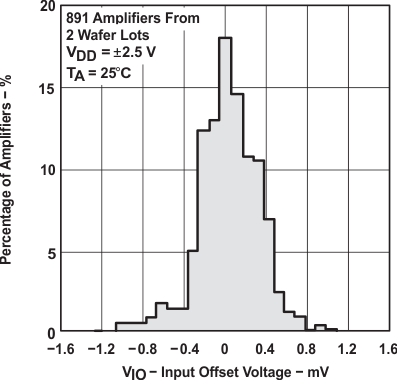Figure 1. Distribution of TLC2272 Input Offset Voltage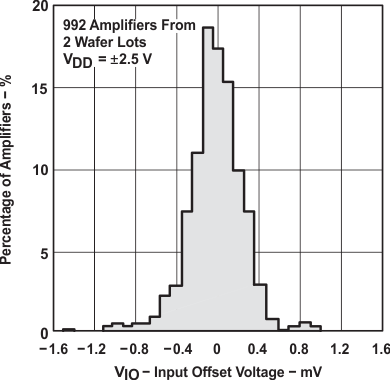Figure 3. Distribution of TLC2274 Input Offset Voltage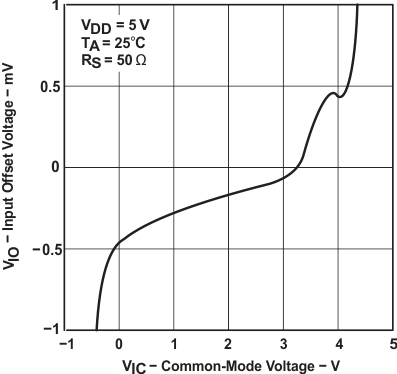Figure 5. Input Offset Voltage vs Common-Mode Voltage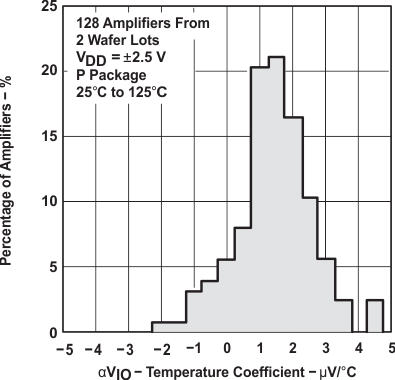Figure 7. Distribution of TLC2272 vs
Input Offset Voltage Temperature CoefficientFigure 9. Distribution of TLC2274 vs
Input Offset Voltage Temperature Coefficient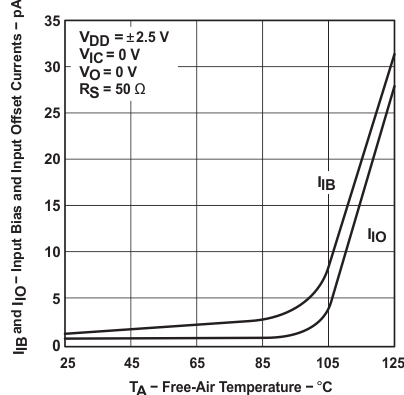Figure 11. Input Bias and Input Offset Current vs
Free-Air Temperature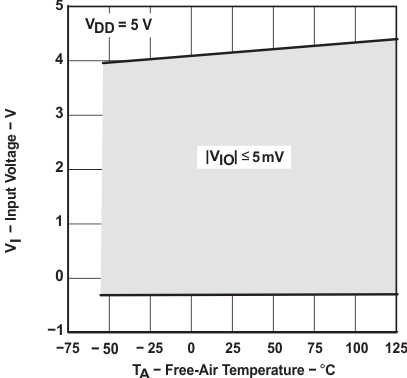Figure 13. Input Voltage vs Free-Air TemperatureFigure 15. Low-Level Output Voltage vs
Low-Level Output Current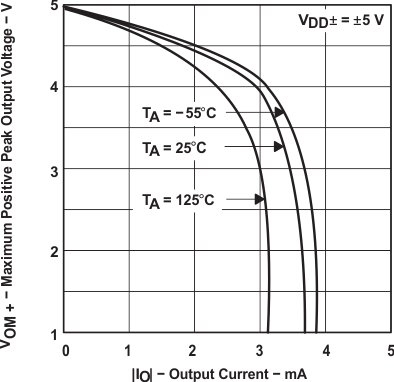Figure 17. Maximum Positive Peak Output Voltage vs
Output Current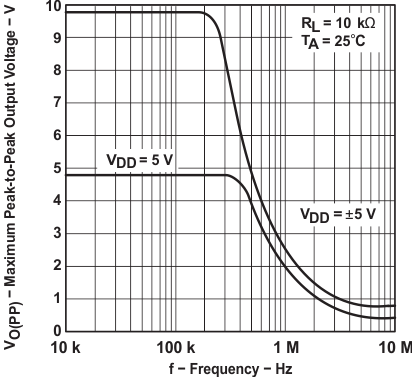Figure 19. Maximum Peak-to-Peak Output Voltage vs
Frequency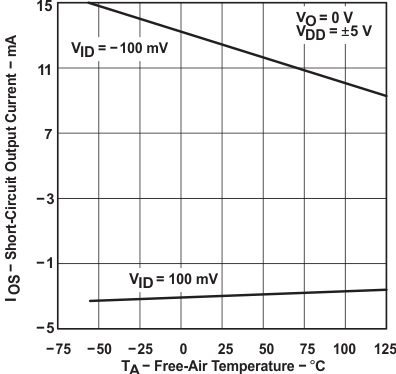Figure 21. Short-Circuit Output Current vs
Free-Air Temperature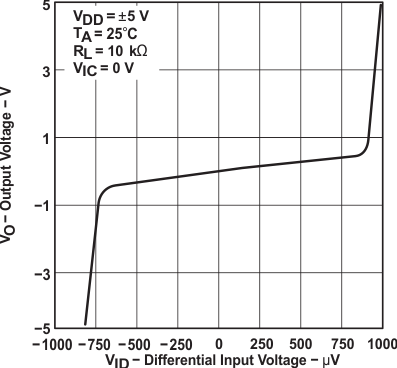Figure 23. Output Voltage vs Differential Input Voltage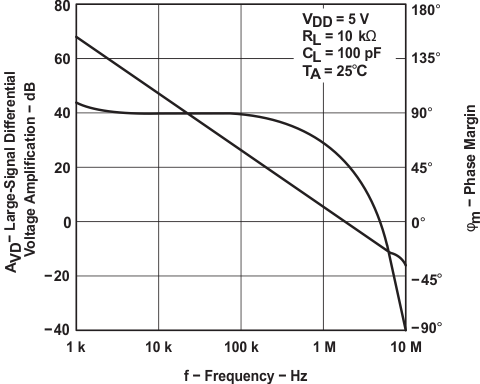Figure 25. Large-Signal Differential Voltage Amplification and Phase Margin vs Frequency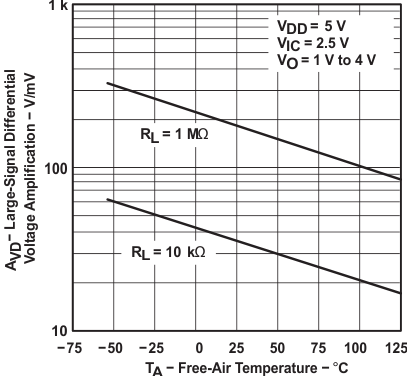Figure 27. Large-Signal Differential Voltage Amplification vs Free-Air Temperature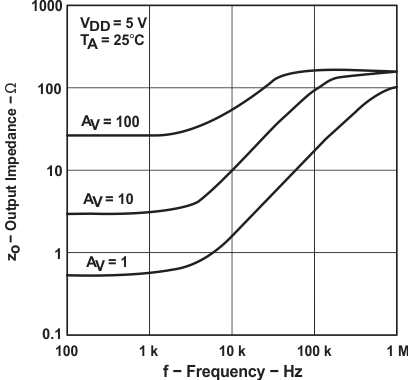Figure 29. Output Impedance vs Frequency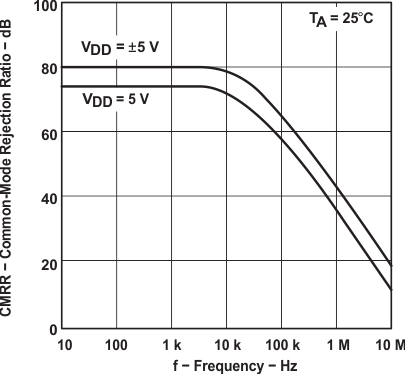Figure 31. Common-Mode Rejection Ratio vs Frequency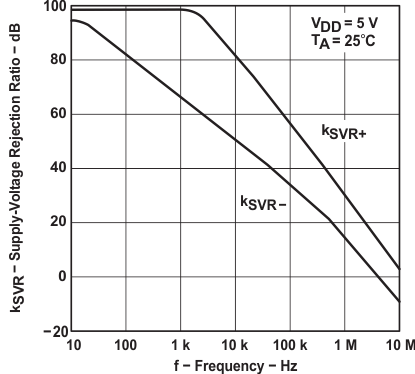Figure 33. Supply-Voltage Rejection Ratio vs Frequency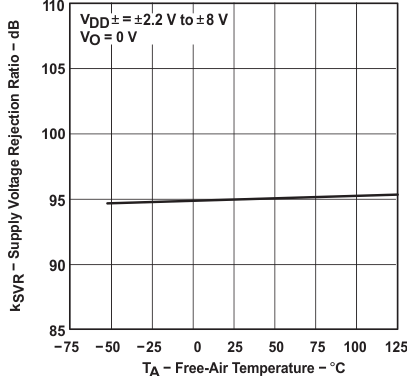Figure 35. Supply-Voltage Rejection Ratio vs
Free-Air Temperature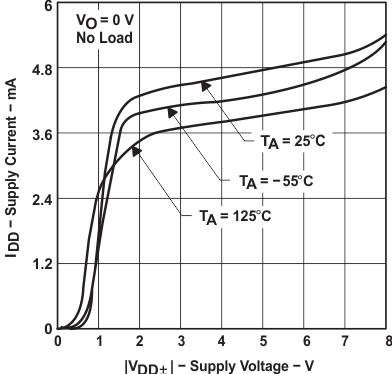Figure 37. TLC2274 Supply Current vs Supply VoltageFigure 39. TLC2274 Supply Current vs Free-Air Temperature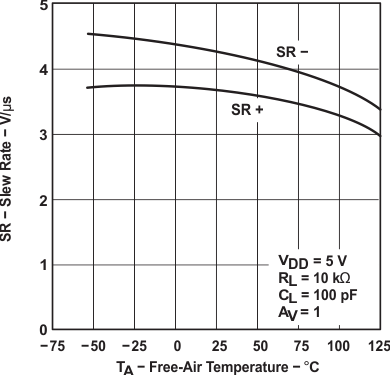Figure 41. Slew Rate vs Free-Air Temperature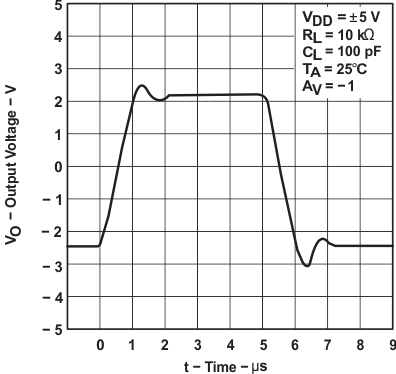Figure 43. Inverting Large-Signal Pulse Response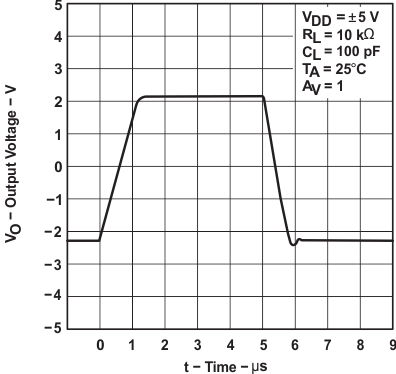Figure 45. Voltage-Follower Large-Signal Pulse Response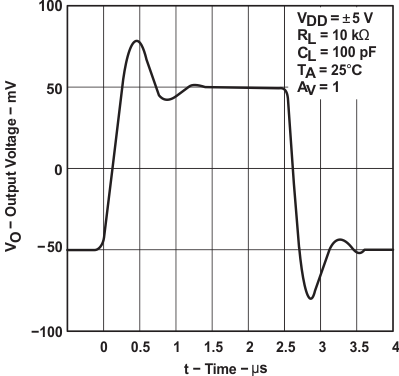Figure 47. Inverting Small-Signal Pulse Response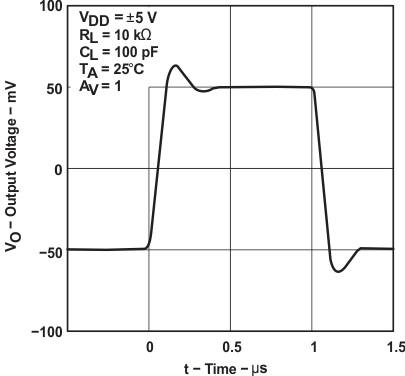Figure 49. Voltage-Follower Small-Signal Pulse Response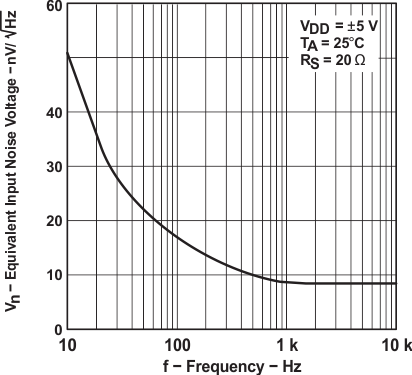Figure 51. Equivalent Input Noise Voltage vs Frequency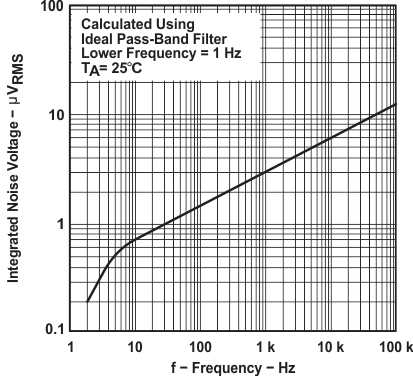Figure 53. Integrated Noise Voltage vs Frequency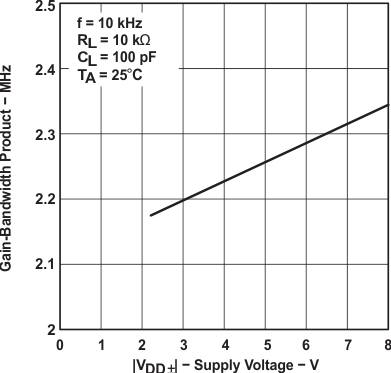Figure 55. Gain-Bandwidth Product vs Supply Voltage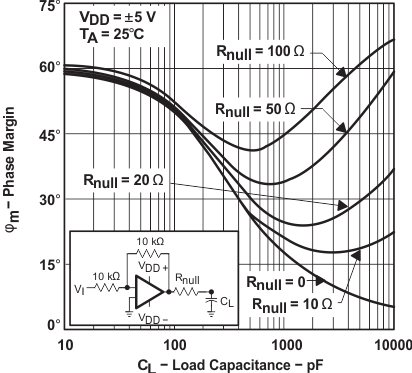Figure 57. Phase Margin vs Load Capacitance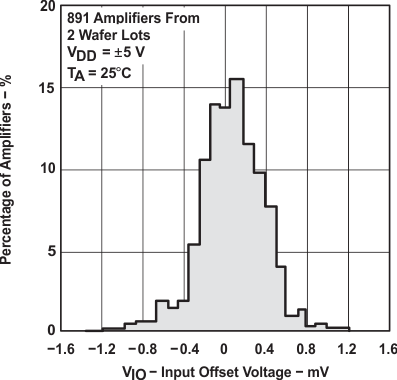Figure 2. Distribution of TLC2272 Input Offset Voltage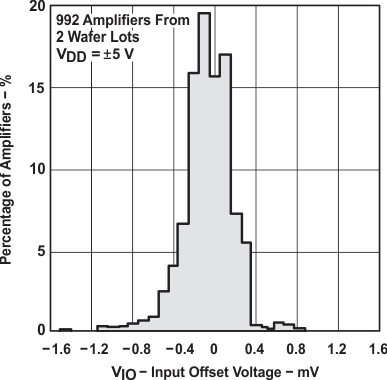Figure 4. Distribution of TLC2274 Input Offset Voltage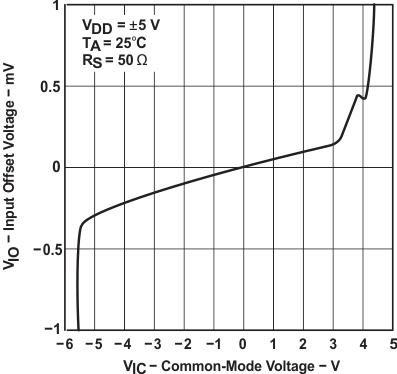Figure 6. Input Offset Voltage vs Common-Mode Voltage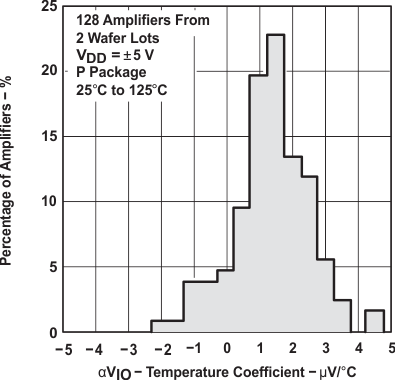Figure 8. Distribution of TLC2272 vs
Input Offset Voltage Temperature Coefficient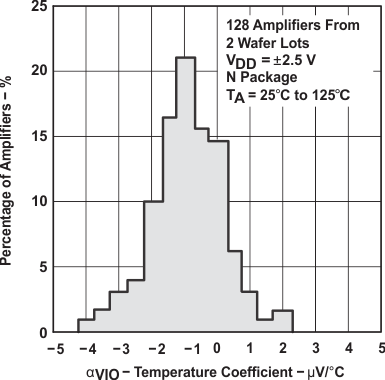Figure 10. Distribution of TLC2274 vs
Input Offset Voltage Temperature Coefficient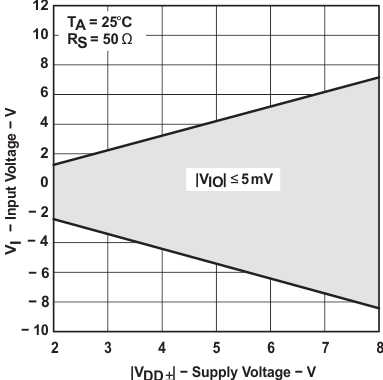Figure 12. Input Voltage vs Supply Voltage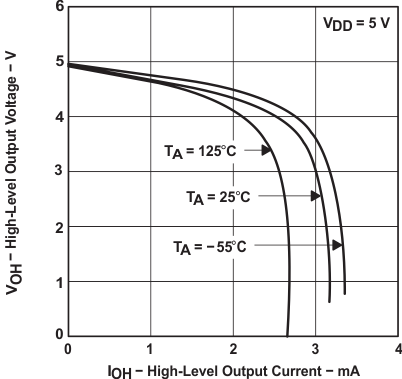Figure 14. High-Level Output Voltage vs
High-Level Output Current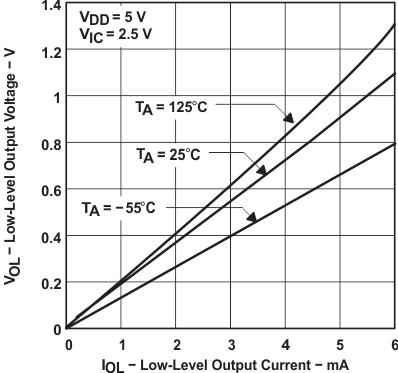Figure 16. Low-Level Output Voltage vs
Low-Level Output Current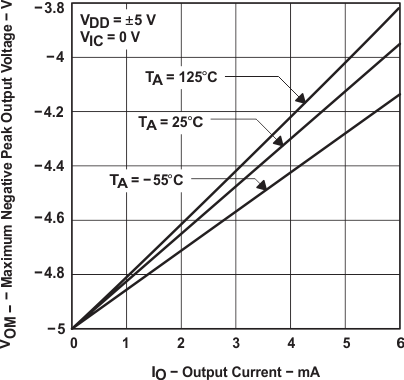Figure 18. Maximum Positive Peak Output Voltage vs
Output Current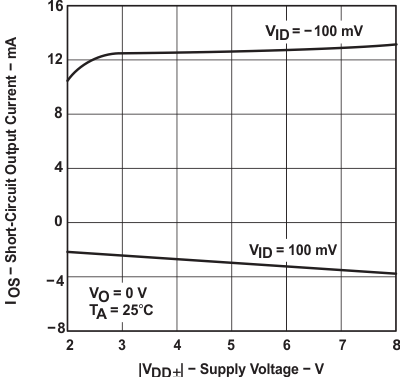Figure 20. Short-Circuit Output Current vs Supply Voltage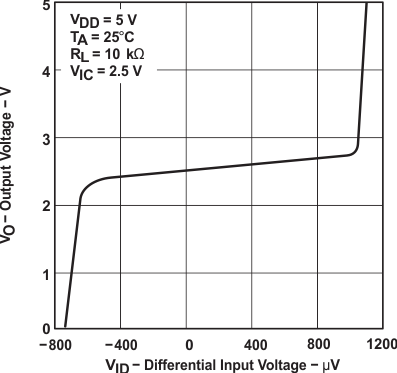Figure 22. Output Voltage vs Differential Input Voltage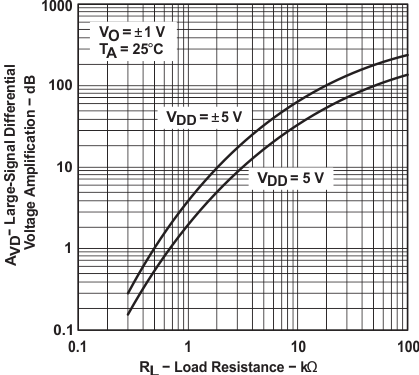Figure 24. Large-Signal Differential Voltage Amplification vs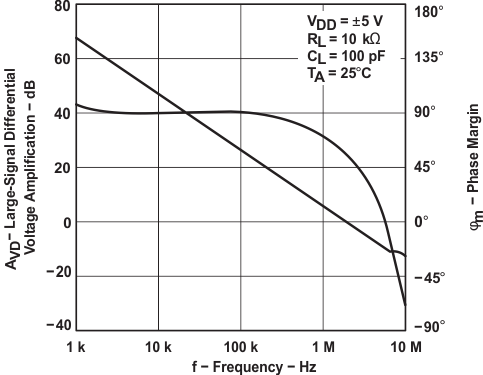Figure 26. Large-Signal Differential Voltage Amplification and Phase Margin vs Frequency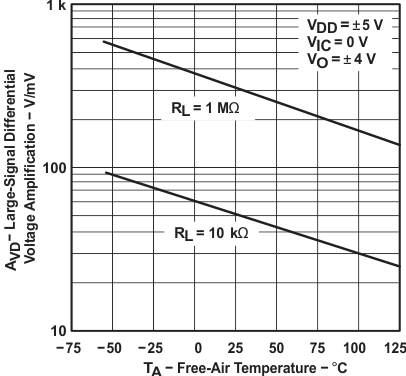Figure 28. Large-Signal Differential Voltage Amplification vs Free-Air Temperature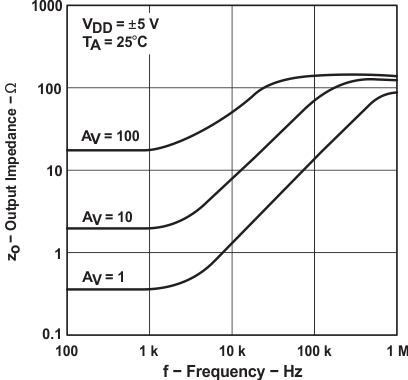Figure 30. Output Impedance vs Frequency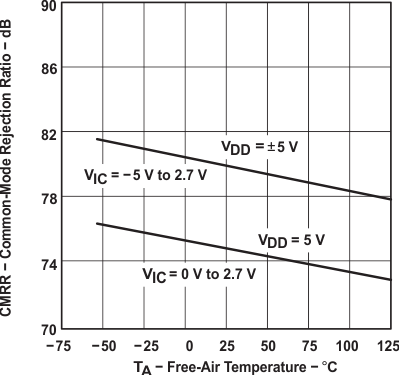Figure 32. Common-Mode Rejection Ratio vs
Free-Air TemperatureFigure 34. Supply-Voltage Rejection Ratio vs Frequency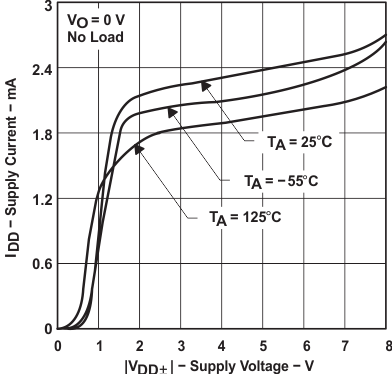Figure 36. TLC2272 Supply Current vs Supply Voltage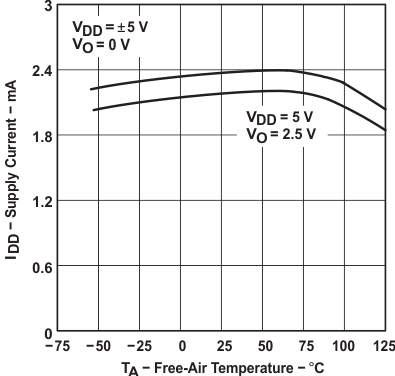Figure 38. TLC2272 Supply Current vs Free-Air Temperature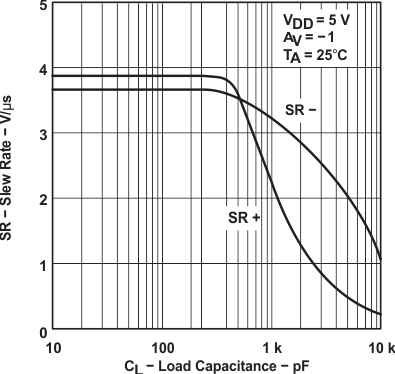Figure 40. Slew Rate vs Load Capacitance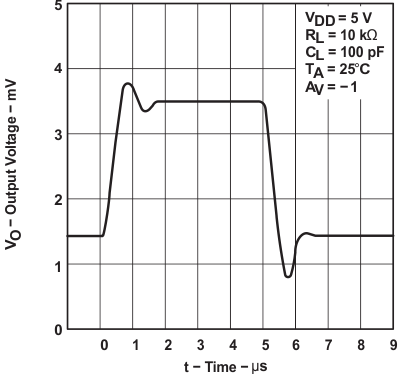Figure 42. Inverting Large-Signal Pulse Response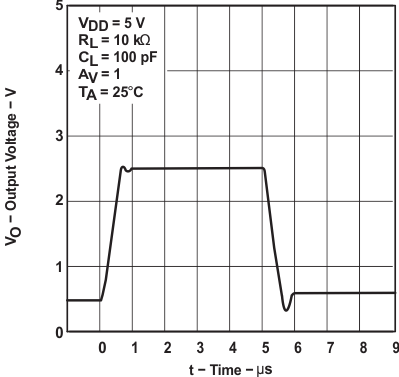Figure 44. Voltage-Follower Large-Signal Pulse Response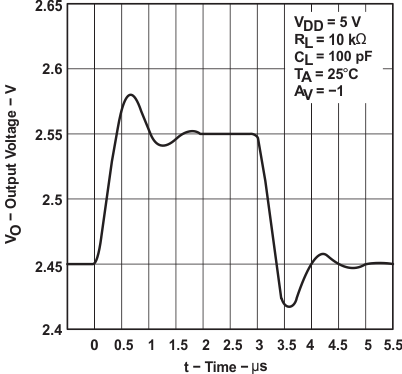Figure 46. Inverting Small-Signal Pulse ResponseFigure 48. Voltage-Follower Small-Signal Pulse Response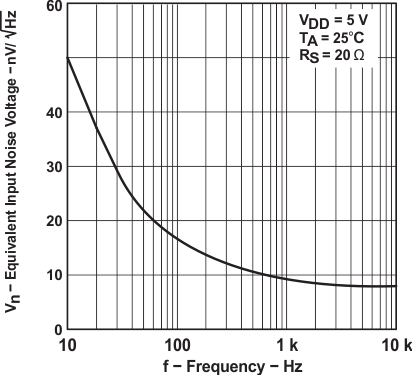Figure 50. Equivalent Input Noise Voltage vs Frequency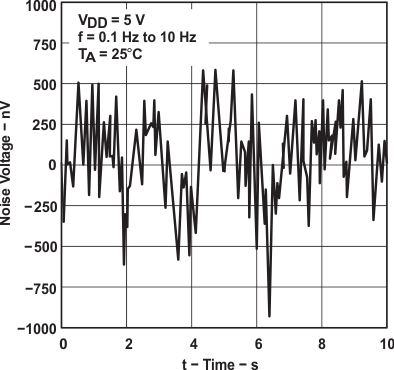Figure 52. Noise Voltage Over a 10 Second Period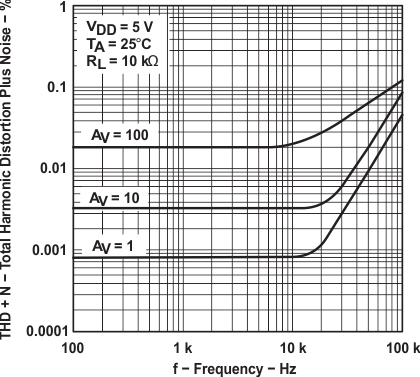Figure 54. Total Harmonic Distortion + Noise vs Frequency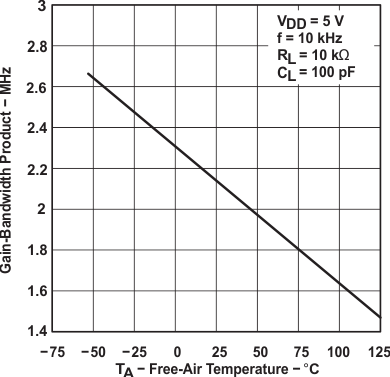Figure 56. Gain-Bandwidth Product vs Free-Air Temperature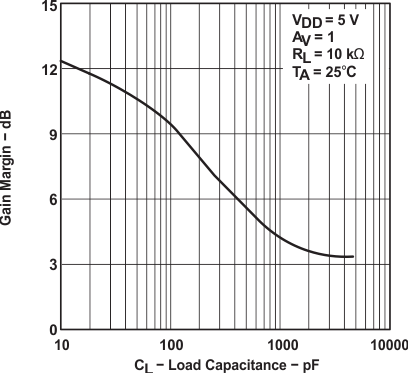Figure 58. Gain Margin vs Load Capacitance